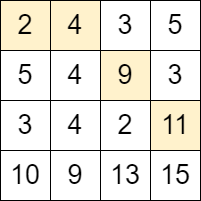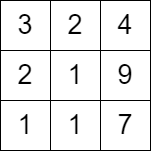2684. Maximum Number of Moves in a Grid
Medium
286
4

You are given a 0-indexed `m x n` matrix `grid` consisting of positive integers.

You can start at any cell in the first column of the matrix, and traverse the grid in the following way:

• From a cell `(row, col)`, you can move to any of the cells: `(row - 1, col + 1)`, `(row, col + 1)` and `(row + 1, col + 1)` such that the value of the cell you move to, should be strictly bigger than the value of the current cell.

Return the maximum number of moves that you can perform.

Example 1:```Input: grid = [[2,4,3,5],[5,4,9,3],[3,4,2,11],[10,9,13,15]]
Output: 3
Explanation: We can start at the cell (0, 0) and make the following moves:
- (0, 0) -> (0, 1).
- (0, 1) -> (1, 2).
- (1, 2) -> (2, 3).
It can be shown that it is the maximum number of moves that can be made.```

Example 2:

```Input: grid = [[3,2,4],[2,1,9],[1,1,7]]
Output: 0
Explanation: Starting from any cell in the first column we cannot perform any moves.
```

Constraints:

• `m == grid.length`
• `n == grid[i].length`
• `2 <= m, n <= 1000`
• `4 <= m * n <= 105`
• `1 <= grid[i][j] <= 106`
Accepted
15.5K
Submissions
34.6K
Acceptance Rate
44.6%

Seen this question in a real interview before?
1/4
Yes
No

Discussion (0)

Related Topics